# Python OpenCV: Meanshift

OpenCV is the huge open-source library for computer vision, machine learning, and image processing and now it plays a major role in real-time operation which is very important in today’s systems. By using it, one can process images and videos to identify objects, faces, or even the handwriting of a human.

#### Meanshift

The idea behind meanshift is that in meanshift algorithm every instance of the video is checked in the form of pixel distribution in that frame. We define an initial window, generally a square or a circle for which the positions are specified by ourself which identifies the area of maximum pixel distribution and tries to keep track of that area in the video so that when the video is running our tracking window also moves towards the region of maximum pixel distribution. The direction of movement depends upon the difference between the center of our tracking window and the centroid of all the k-pixels inside that window.

Meanshift is a very useful method to keep track of a particular object inside a video. Meanshift can separate the static background of a video and the moving foreground object.

Examples:
1.The tracking windows is tracking the football.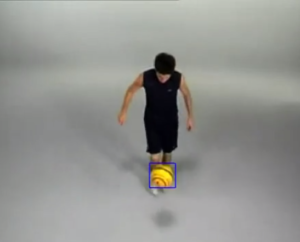2.The tracking window is tracking the juggling ball.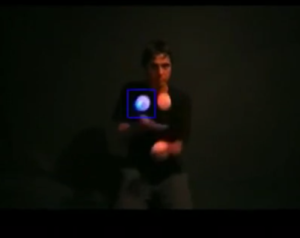3.The tracking window is tracking the football player.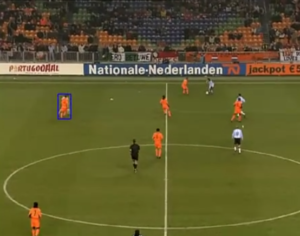`# Python porgram to demonstrate ` `# meanshift  ` ` `  ` `  `import` `numpy as np ` `import` `cv2 ` `  `  ` `  `# read video ` `cap ``=` `cv2.VideoCapture(``'sample.mp4'``) ` `  `  `# retrieve the very first  ` `# frame from the video ` `_, frame ``=` `cap.read() ` `  `  `# set the region for the ` `# tracking window p, q, r, s ` `# put values according to yourself ` `p, q, r, s ``=` `150``, ``150``, ``460``, ``100` `track_window ``=` `(r, p, s, q) ` `  `  `     `  `# create the region of interest ` `r_o_i ``=` `frame[p:p ``+` `q, r:r ``+` `s] ` ` `  `# converting BGR to HSV format ` `hsv ``=` `cv2.cvtColor(frame, cv2.COLOR_BGR2HSV) ` `  `  `# apply mask on the HSV frame ` `mask ``=` `cv2.inRange(hsv,  ` `                   ``np.array((``0.``, ``61.``, ``33.``)), ` `                   ``np.array((``180.``, ``255.``, ``255.``))) ` ` `  `# get histogram for hsv channel ` `roi ``=` `cv2.calcHist([hsv], [``0``], mask,  ` `                   ``[``180``], [``0``, ``180``]) ` ` `  `# normalize the retrieved values ` `cv2.normalize(roi, roi, ``0``, ``255``,  ` `              ``cv2.NORM_MINMAX) ` `  `  `# termination criteria, either 15  ` `# iteration or by at least 2 pt ` `termination ``=` `(cv2.TERM_CRITERIA_EPS |  ` `               ``cv2.TERM_CRITERIA_COUNT ` `               ``, ``15``, ``2` `) ` `  `  `while``(``True``): ` `    ``_, frame ``=` `cap.read() ` `  `  `    ``frame ``=` `cv2.resize(frame,  ` `                       ``(``1280``, ``720``),  ` `                       ``fx ``=` `0``,  ` `                       ``fy ``=` `0``,  ` `                       ``interpolation ``=` `cv2.INTER_CUBIC) ` `  `  `    ``# convert BGR to HSV format ` `    ``hsv ``=` `cv2.cvtColor(frame,  ` `                       ``cv2.COLOR_BGR2HSV) ` `     `  `    ``bp ``=` `cv2.calcBackProject([hsv],  ` `                             ``[``0``],  ` `                             ``roi,  ` `                             ``[``0``, ``180``],  ` `                             ``1``) ` `  `  `    ``# applying meanshift to get the new region ` `    ``_, track_window ``=` `cv2.meanShift(bp,  ` `                                    ``track_window,  ` `                                    ``termination) ` `  `  `    ``# Draw track window on the frame ` `    ``x, y, w, h ``=` `track_window ` `    ``vid ``=` `cv2.rectangle(frame, (x, y),  ` `                        ``(x ``+` `w, y ``+` `h),  ` `                        ``255``, ``2``) ` `     `  `    ``# show results ` `    ``cv2.imshow(``'tracker'``, vid) ` `  `  `    ``k ``=` `cv2.waitKey(``1``) & ``0xff` `    ``if` `k ``=``=` `ord``(``'q'``): ` `        ``break` `         `  `# release cap object ` `cap.release() ` ` `  `# destroy all opened windows ` `cv2.destroyAllWindows() `

Output: Some frames from the ouput video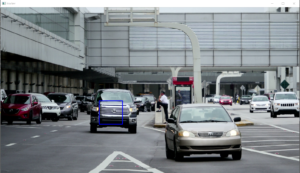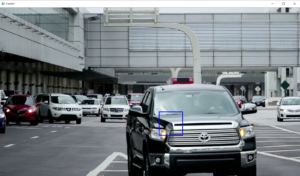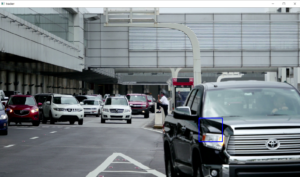There are 2 main disadvantages of using the Meanshift for object tracking.

• The size of the tracking window remains the same irrespective of the distance of the object from the camera.
• The Window will track the object only when it is in the region of that object. So we must hardcode our position of the window carefully.

My Personal Notes arrow_drop_upCheck out this Author's contributed articles.

If you like GeeksforGeeks and would like to contribute, you can also write an article using contribute.geeksforgeeks.org or mail your article to contribute@geeksforgeeks.org. See your article appearing on the GeeksforGeeks main page and help other Geeks.

Please Improve this article if you find anything incorrect by clicking on the "Improve Article" button below.

Article Tags :

Be the First to upvote.

Please write to us at contribute@geeksforgeeks.org to report any issue with the above content.